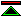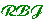# Computing with Reals - motivation

It is not possible using a digital computer to represent arbitrary real numbers. A subset of reals called computable reals are representable using algorithms. Given a suitable means of representing computable reals (sometimes known as an admissible representation, see [Weihrauch95b]) a wide range of functions from analysis can be effectively computed, probably all those which are shown to be constructive in [Bishop85].

## Why Bother?

The first place to look for reasons is to analysis itself. The real numbers were introduced because many problems do not have exact solutions in any more restricted number system. In effect a real number may be thought of as a sequence of ever more precise rational approximations to a problem whose solution can be positioned in the rational line but which does not coincide with any rational number.

Computation using reals of this kind has the advantage over computation with arbitrary precision floating point numbers (or rationals), that the precision of the final result can be selected and known. With floating point numbers there is no general method for determining the precision of arithmetic required to achieve any particular level of precision in the end result.

A second advantage is that this kind of real number is suitable for use in formal theorem proving environment, because the result of a computation can be expressed as a true (if complex) equation, and the numbers operated on have the same algebraic properties as the real numbers (because they are real numbers). When operating with floating point numbers rounding errors cause the algebraic properties of the number system to be messed up, and the result of a floating point computation is often not an arithmetic truth, but a cock-up. If a computation is not only imprecise, but is not even within any known error bound, then it forms the basis for no meaningful claim which might be demontrated in a proof system.©created 1996-02-03 modified 2012-09-29